# Quiz on Flow Measurement

Q1. Which of the following is the definition  of viscosity?

1. A measure of a fluid’s tendency to resist flow
2. A measure of specific gravity of a fluid
3. The density of a fluid per unit of volume
4. A measure of dielectric constant a fluid

Q2. Which of the following are variables in the Reynolds number equation?  Select all options that apply.

1. Fluid density
2. Fluid velocity
3. Fluid temperature
4. Pipe inside diameter
5. Fluid viscosity

Q3.   Which of the following is the appropriate definition of rangeability?

1. Maximum flow rate that a flow meter is capable of reading
2. Ratio of maximum to minimum flow rate under which a flow meter can maintain measurement accuracy
3. Maximum flow rate that occurs in the process flow of a particular application
4. The difference between the upper range limit and the lower range limit of a flow meter.

Q4. When using the orifice plate for flow measurement, the fluid flowing through the orifice will create a _____________ drop.

1. Voltage
2. Velocity
3. Pressure

Q5. The pressure of the fluid is ____________ at the upstream of the orifice plate.

1. Higher
2. Lower
3. Uniform

Q6. The two types of pressure created and measured in a pitot tube are static pressure and ______________ pressure.

1. Impact
2. Vacuum
3. Atmospheric
4. Absolute

Q7. Rotameters must be mounted __________.

1. Horizontally
2. Vertically
3. Diagonally

Q8. Which of the following differential pressure flow meter has high pressure loss ?

1. Venturi
2. Orifice
3. Nozzle
4. Pitot

Q9. Time-of-flight ultrasonic flow meters operate on the principle that the time taken by the ultrasonic sound wave will _____________ (increase/decrease)  when directed with flow and ________________(increase/decrease) when directed against flow.

Q10. Magnetic flow meters measure flow rate based on Faraday’s law of induction. ( True or false)

1. True
2. False

Q11. If the Reynolds number is <2000 the flow profile will be ____LAMINAR_______  and for turbulent  flow profile the Reynolds number will be ____>4000_____.

Q12. As fluid passes through a bluff body, it separates and generates small eddies and the frequency this eddies is proportional to fluid velocity. Which flow meter uses this principle ?

1. Vortex
2. Turbine
3. Positive displacement
4. Ultrasonic

Q13. What is the purpose of providing flow straightners upstream of a flow meter ?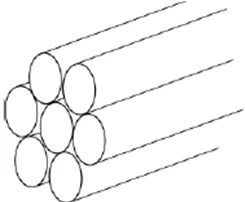ANS: TO AVOID TURBULENT FLOW

Q14.  What is  the uncertainty in the flow measurement of a flowmeter having an accuracy of  1% of flow rate when the flow rate is (i) 500 gpm and (ii) 10 gpm.

Q15. For a flow meter, what is application maximum and what is meter maximum?

Q16. Volumetric flow rate = __________V_________ ×  A.

Q17. Give one unit of mass flow rate.

ANS:  kg/hr,  lit/hr

Q19.  Identify the type of orifice plates ( eccentric/ segmental/ concentric).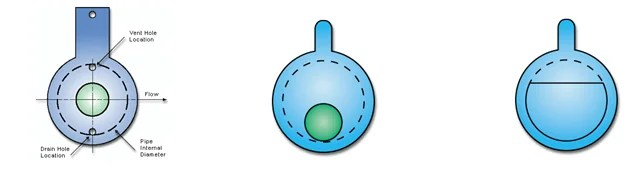_______CONCENTRIC________   ECENTRIC         _______SEGMENTAL___

Q20. Show the           Vena contracta point in the pressure profile shown below.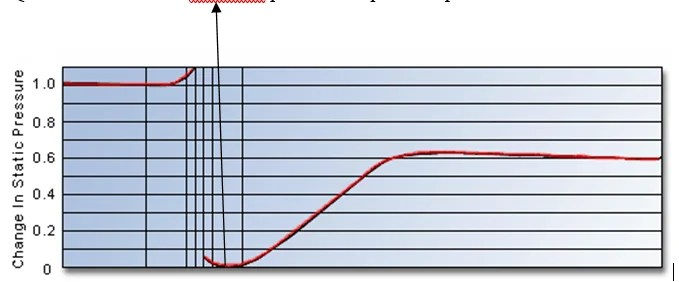Q21. A small drain hole is sometimes drilled in an orifice plate. What is the purpose?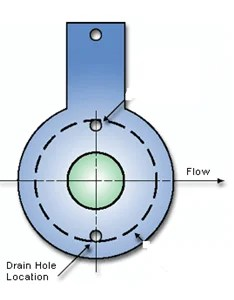Q22. Give four primary element flow meter errors.

• ___________________________
• ___________________________
• ___________________________
• ___________________________

Q23. Give the name of a flow meter which measures mass flow rate.

ANS: 1. CORILIS MASS FLOW MEASUREMENT

1. THERMAL MASS FLOW MEASUREMENT

Q24. The flow rate through a pipe is 125 ft3/sec. If  area A1 is 25 ft2 and velocity V1 is 5 ft/sec, calculate the velocity V2 if area A2 is 12.5 ft2 ?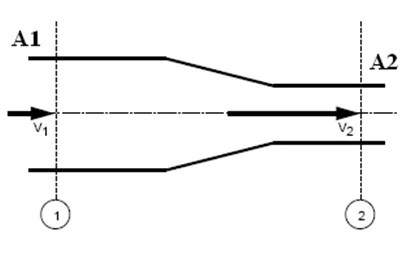V1A1=V2A2

V2=V1A1/A2

Q25. Match each flow meter class to the corresponding description given.

(1) Velocity                                         ___B__

(2) Positive displacement                   ___C__

(3) Differential producers                   __D___

(4) Mass                                              ___A__

(A) Measures the actual weight of a fluid as it is transported through a pipe per unit of time; not affected by changes in volume or density.

(B) Works by producing an output based upon the speed of a fluid flowing past a  stationary point that is linear to volumetric flow rate.

(C) Measures volumetric flow rate directly by repeatedly trapping and measuring a sample of process fluid.

(D) Creates high–pressure and low–pressure areas in the process flow by introducing an obstruction in the flow path. Volumetric flow rate is then calculated using Bernoulli’s equation.

paktechpoint.com

### 1 Response

1.ปั้มไลค์ says:

Like!! I blog frequently and I really thank you for your content. The article has truly peaked my interest.

error: tected !!Content is pro